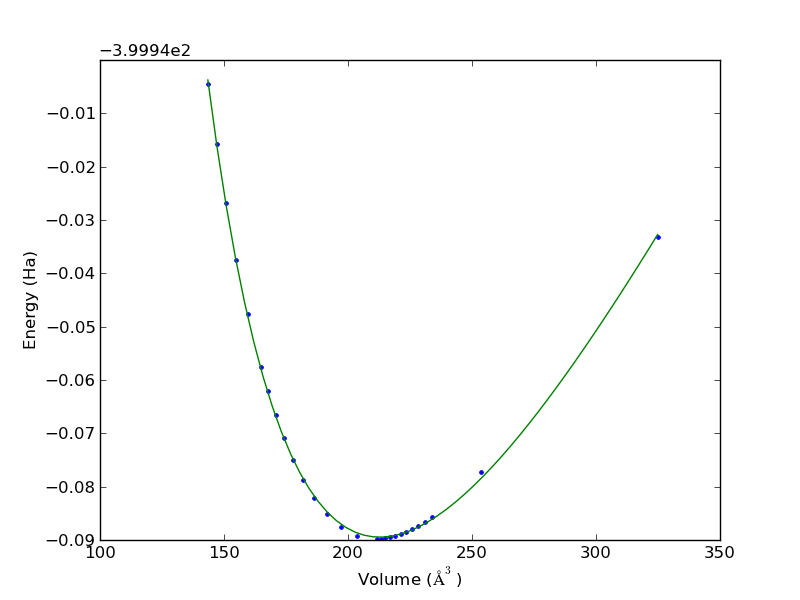## Estimating uncertainties in equations of state

| categories: uncategorized | tags: | View Comments

We often use DFT to calculate the energy of a unit cell as a function of volume. Then, we fit an equation of state to the data to estimate the volume that minimizes the total energy, and the bulk modulus of the material. 10.1016/j.comphys.2003.12.001

volume energy
324.85990899 -399.9731688470
253.43999457 -400.0172393178
234.03826687 -400.0256270548
231.12159387 -400.0265690700
228.40609504 -400.0273551120
225.86490337 -400.0280030862
223.47556626 -400.0285313450
221.21992353 -400.0289534593
219.08319566 -400.0292800709
217.05369547 -400.0295224970
215.12089909 -400.0296863867
213.27525144 -400.0297809256
211.51060823 -400.0298110000
203.66743321 -400.0291665573
197.07888649 -400.0275017142
191.39717952 -400.0250998136
186.40163591 -400.0221371852
181.94435510 -400.0187369863
177.92077043 -400.0149820198
174.25380090 -400.0109367042
170.88582166 -400.0066495100
167.76711189 -400.0021478258
164.87096104 -399.9974753449
159.62553397 -399.9876885136
154.97005460 -399.9774175487
150.78475335 -399.9667603369
146.97722201 -399.9557686286
143.49380641 -399.9445262604
import numpy as np
import matplotlib.pyplot as plt
from pycse import nlinfit

# data
V = np.array([row for row in data])
E = np.array([row for row in data])

plt.plot(V, E, '.')
plt.xlabel('Volume ($\AA^3$)')
plt.ylabel('Energy (Ha)')

def Murnaghan(vol, E0, B0, BP, V0):
'''
given a vector of parameters and volumes, return a vector of energies.
equation From PRB 28,5480 (1983)
'''

E = E0 + B0*vol/BP*(((V0/vol)**BP)/(BP-1)+1) - V0*B0/(BP-1.)

return E

guess = [-400, 0.5, 2, 210]
pars, pint, SE = nlinfit(Murnaghan, V, E, guess, alpha=0.05)
E0, B0, BP, V0 = pint

Vfit = np.linspace(V.min(), V.max())
plt.plot(Vfit, Murnaghan(Vfit, *pars))
plt.savefig('images/eos-uncertainty.png')

print '95% confidence intervals'
print 'V0 = {0} bohr**3'.format(V0)
print 'E0 = {0} Ha'.format(E0)
print 'B0 = {0} GPA'.format([x * 29421.010901602753 for x in B0])

95% confidence intervals
V0 = [212.27788154532402, 213.27897592511891] bohr**3
E0 = [-400.0297027767362, -400.02922937100408] Ha
B0 = [108.62283904402159, 111.20447706313001] GPAYou can see the fit is not perfect, and there is corresponding uncertainty in the estimated parameters. A nice feature of the Murnaghan equation of state is that the parameters are directly the quantities of interest, so the uncertainties are directly calculated here. For other models, e.g. a polynomial fit, you would have to propagate the errors in the parameters to the properties.